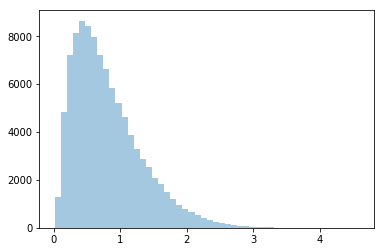# Branching for numerical stability

To make a transformed distribution I want to implement an inverse softplus function `log(exp(x)-1)`.
To do this i would need to implement something like this

``````if x > 20:
return x
else:
return (x.exp() - 1).log()
``````

`torch.nn.functional.threshold` would be promising, except it doesn’t support tensors in its `value` argument.

`torch.where` looks good too, but I would prefer to have a `0.3` compatible solution.

The `cond * (...) + (1-cond) * (...)` hack used in the below thread doesn’t work here since the `(...)`'s may be infinite.

Thanks!

You could clip the term somewhere outside the range and then multiply, even if it isn’t the most efficient solution.

Best regards

Thomas

Thanks! That’s a good idea.

For the record, I ended up installing pytorch 0.4, which was actually a delight to compile.
Here’s the code:

``````import torch
import torch.nn as nn
from torch.distributions import constraints
from torch.distributions.transforms import Transform

class SoftplusTransform(Transform):
r"""
Transform via the mapping :math:`y = \log(1 + \exp(x))`
"""
domain = constraints.real
codomain = constraints.positive
bijective = True
sign = +1

def __init__(self):
super().__init__()
self.softplus = nn.Softplus()
self.threshold = 20
self.log_sigmoid = nn.LogSigmoid()

def _call(self, x):
return self.softplus(x)

def _inverse(self, y):

def log_abs_det_jacobian(self, x, y):
return self.log_sigmoid(x)
``````
``````import pyro.distributions as dist
import seaborn as sns
sns.distplot(
dist.TransformedDistribution(dist.Normal(0,1), SoftplusTransform()).sample(sample_shape=(100000,)).data.numpy(),
kde=False)
``````1 Like